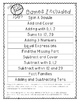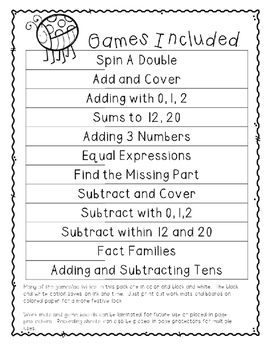# No Prep Math Games - AprilSubject
Resource Type
File Type
PDF (5 MB|49 pages)
Standards
\$2.50
• Product Description
• Standards
This pack includes 12 no prep math games to enjoy during the the month of April. The games can be printed in color or black and white. Games can also be laminated or put in page protectors for multiple uses.

Games included:
1. Spin a Double
2. Add and Cover (Sums to 12, 20, 35)
4. Sums to 12 and 20 (writing number sentences)
5. Equal Expressions
7. Find the Missing Part
8. Subtract and Cover
9. Subtraction with 0,1,2
10. Subtraction within 10 and 20
11. Fact Families
Determine the unknown whole number in an addition or subtraction equation relating three whole numbers. For example, determine the unknown number that makes the equation true in each of the equations 8 + ? = 11, 5 = ▯ - 3, 6 + 6 = ▯.
Understand the meaning of the equal sign, and determine if equations involving addition and subtraction are true or false. For example, which of the following equations are true and which are false? 6 = 6, 7 = 8 - 1, 5 + 2 = 2 + 5, 4 + 1 = 5 + 2.
Apply properties of operations as strategies to add and subtract. If 8 + 3 = 11 is known, then 3 + 8 = 11 is also known. (Commutative property of addition.) To add 2 + 6 + 4, the second two numbers can be added to make a ten, so 2 + 6 + 4 = 2 + 10 = 12. (Associative property of addition.)
Total Pages
49 pages